Setup for 5 Player a Game of Food Chain Magnate

Originally generated on 1/11/2019 11:17:35 AM

Player Setup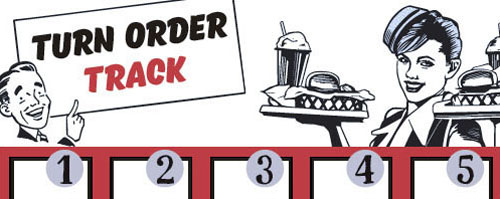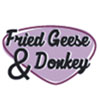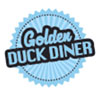Other Setup

Bank starts with \$250.00
Number of 1x Employee cards used = 3

Map (Random)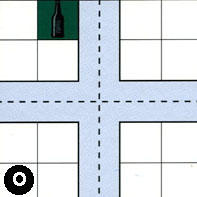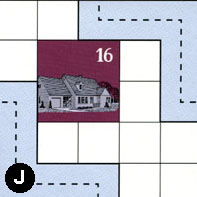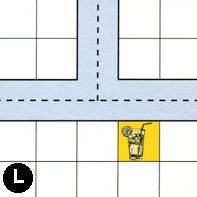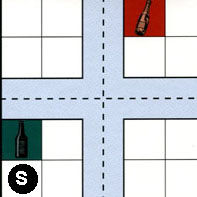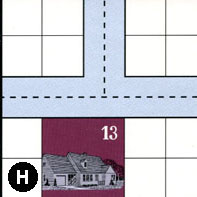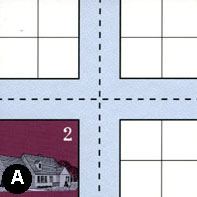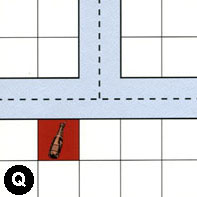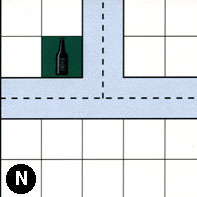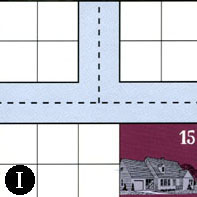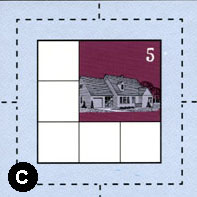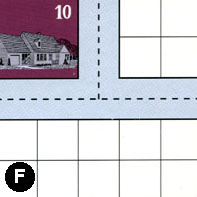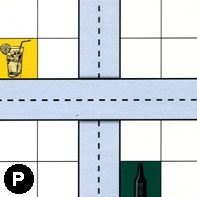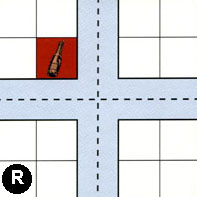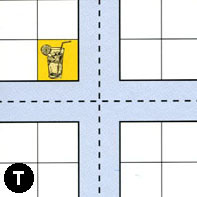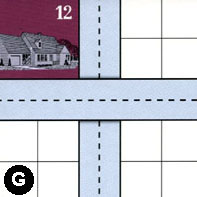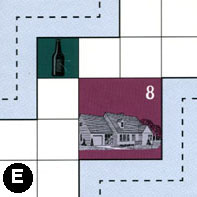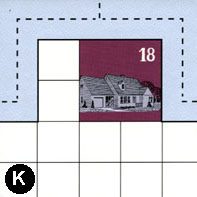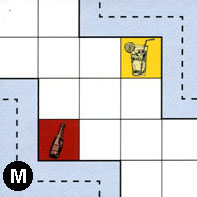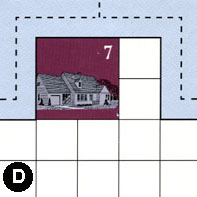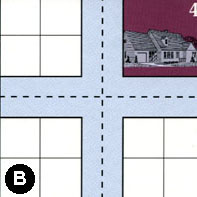Map Stats

Map Option = Random
Total Number of Tiles = 20
Total Number of Starting Houses = 11
Total Number of Beer Spots = 5
Total Number of Soda Spots = 4
Total Number of Lemonade Spots = 4
Total Number of Drink Spots = 13
Number of independent path systems = 2
Number of independent neighborhoods = 18

Path System 1
Size = Large
Number of Tiles = 20
Number of Paths = 75
Contains Loop(s) = No
Number of Starting Houses = 11
Starting Houses Pct of Map (%) = 100
Number of Beer Spots = 5
Number of Soda Spots = 4
Number of Lemonade Spots = 4
Number of Drink Spots = 13
Drink Spots Pct of Map (%) = 100
Tile Ids = A, B, C, D, E, F, G, H, I, J, K, L, M, N, O, P, Q, R, S, T
Paths = OWN, JEN, ONE, AWN, AWE, QWE, NWN, NNE, NWE, QWN, PWE, KWN, MWS, RWN, PNS, RNE, RWE, RWS, TWN, DWE, DWN, MEN, DNE, TWE, IWE, SWN, LWE, JWS, LWN, LNE, SNE, SWE, IWN, CWN, HWN, SWS, SNS, SES, HNE, HWE, CNE, CWE, INE, TNE, RNS, RES, TNS, GWE, TWS, TES, CWS, GNS, BWN, BWE, BNE, BNS, BWS, BES, CNS, CES, KNE, KWE, QNE, ANE, OWE, OWS, ONS, OES, ANS, FWE, EWN, FWN, FNE, AWS, AES What do these mean?
Starting House Ids = 0, 2, 4, 5, 7, 10, 12, 13, 15, 16, 18
Path System 2
Size = Tiny
Number of Tiles = 1
Number of Paths = 1
Contains Loop(s) = No
Number of Starting Houses = 1
Starting Houses Pct of Map (%) = 9
Number of Beer Spots = 0
Number of Soda Spots = 0
Number of Lemonade Spots = 0
Number of Drink Spots = 0
Drink Spots Pct of Map (%) = 0
Tile Ids = E
Paths = EES What do these mean?
Starting House Ids = 8

Neighborhood 1
Total Size = Small
Number of Total Spaces = 4
Number of Empty Spaces (for new houses & gardens) = 4
Number of Beer Spots = 0
Number of Soda Spots = 0
Number of Lemonade Spots = 0
Number of Drink Spots = 0
Number of Starting Houses = 0
Starting Houses Pct of Map (%) = 0
Tile Ids = O
Neighborhood 2
Total Size = Huge
Number of Total Spaces = 99
Number of Empty Spaces (for new houses & gardens) = 86
Number of Beer Spots = 2
Number of Soda Spots = 1
Number of Lemonade Spots = 2
Number of Drink Spots = 5
Number of Starting Houses = 2
Starting Houses Pct of Map (%) = 18
Tile Ids = A, I, J, L, N, O, P, Q, R, S, T
Starting House Ids = 15, 16
Neighborhood 3
Total Size = Small
Number of Total Spaces = 4
Number of Empty Spaces (for new houses & gardens) = 4
Number of Beer Spots = 0
Number of Soda Spots = 0
Number of Lemonade Spots = 0
Number of Drink Spots = 0
Number of Starting Houses = 0
Starting Houses Pct of Map (%) = 0
Tile Ids = L
Neighborhood 4
Total Size = Medium
Number of Total Spaces = 8
Number of Empty Spaces (for new houses & gardens) = 7
Number of Beer Spots = 0
Number of Soda Spots = 1
Number of Lemonade Spots = 0
Number of Drink Spots = 1
Number of Starting Houses = 0
Starting Houses Pct of Map (%) = 0
Tile Ids = L, S
Neighborhood 5
Total Size = Medium
Number of Total Spaces = 8
Number of Empty Spaces (for new houses & gardens) = 8
Number of Beer Spots = 0
Number of Soda Spots = 0
Number of Lemonade Spots = 0
Number of Drink Spots = 0
Number of Starting Houses = 0
Starting Houses Pct of Map (%) = 0
Tile Ids = H, S
Neighborhood 6
Total Size = Medium
Number of Total Spaces = 10
Number of Empty Spaces (for new houses & gardens) = 6
Number of Beer Spots = 0
Number of Soda Spots = 0
Number of Lemonade Spots = 0
Number of Drink Spots = 0
Number of Starting Houses = 1
Starting Houses Pct of Map (%) = 9
Tile Ids = H
Starting House Ids = 13
Neighborhood 7
Total Size = Medium
Number of Total Spaces = 8
Number of Empty Spaces (for new houses & gardens) = 8
Number of Beer Spots = 0
Number of Soda Spots = 0
Number of Lemonade Spots = 0
Number of Drink Spots = 0
Number of Starting Houses = 0
Starting Houses Pct of Map (%) = 0
Tile Ids = A, O
Neighborhood 8
Total Size = Medium
Number of Total Spaces = 12
Number of Empty Spaces (for new houses & gardens) = 11
Number of Beer Spots = 1
Number of Soda Spots = 0
Number of Lemonade Spots = 0
Number of Drink Spots = 1
Number of Starting Houses = 0
Starting Houses Pct of Map (%) = 0
Tile Ids = H, I, S
Neighborhood 9
Total Size = Medium
Number of Total Spaces = 9
Number of Empty Spaces (for new houses & gardens) = 5
Number of Beer Spots = 0
Number of Soda Spots = 0
Number of Lemonade Spots = 0
Number of Drink Spots = 0
Number of Starting Houses = 1
Starting Houses Pct of Map (%) = 9
Tile Ids = C
Starting House Ids = 5
Neighborhood 10
Total Size = Medium
Number of Total Spaces = 8
Number of Empty Spaces (for new houses & gardens) = 4
Number of Beer Spots = 0
Number of Soda Spots = 0
Number of Lemonade Spots = 0
Number of Drink Spots = 0
Number of Starting Houses = 1
Starting Houses Pct of Map (%) = 9
Tile Ids = A, F
Starting House Ids = 2
Neighborhood 11
Total Size = Large
Number of Total Spaces = 61
Number of Empty Spaces (for new houses & gardens) = 47
Number of Beer Spots = 2
Number of Soda Spots = 0
Number of Lemonade Spots = 0
Number of Drink Spots = 2
Number of Starting Houses = 3
Starting Houses Pct of Map (%) = 27
Tile Ids = A, E, F, K, P, Q
Starting House Ids = 8, 10, 18
Neighborhood 12
Total Size = Medium
Number of Total Spaces = 12
Number of Empty Spaces (for new houses & gardens) = 12
Number of Beer Spots = 0
Number of Soda Spots = 0
Number of Lemonade Spots = 0
Number of Drink Spots = 0
Number of Starting Houses = 0
Starting Houses Pct of Map (%) = 0
Tile Ids = G, I, T
Neighborhood 13
Total Size = Small
Number of Total Spaces = 4
Number of Empty Spaces (for new houses & gardens) = 4
Number of Beer Spots = 0
Number of Soda Spots = 0
Number of Lemonade Spots = 0
Number of Drink Spots = 0
Number of Starting Houses = 0
Starting Houses Pct of Map (%) = 0
Tile Ids = G
Neighborhood 14
Total Size = Medium
Number of Total Spaces = 8
Number of Empty Spaces (for new houses & gardens) = 7
Number of Beer Spots = 0
Number of Soda Spots = 1
Number of Lemonade Spots = 0
Number of Drink Spots = 1
Number of Starting Houses = 0
Starting Houses Pct of Map (%) = 0
Tile Ids = P, R
Neighborhood 15
Total Size = Medium
Number of Total Spaces = 23
Number of Empty Spaces (for new houses & gardens) = 20
Number of Beer Spots = 0
Number of Soda Spots = 1
Number of Lemonade Spots = 2
Number of Drink Spots = 3
Number of Starting Houses = 0
Starting Houses Pct of Map (%) = 0
Tile Ids = M, R, T
Neighborhood 16
Total Size = Large
Number of Total Spaces = 32
Number of Empty Spaces (for new houses & gardens) = 28
Number of Beer Spots = 0
Number of Soda Spots = 0
Number of Lemonade Spots = 0
Number of Drink Spots = 0
Number of Starting Houses = 1
Starting Houses Pct of Map (%) = 9
Tile Ids = B, D, G, T
Starting House Ids = 7
Neighborhood 17
Total Size = Medium
Number of Total Spaces = 8
Number of Empty Spaces (for new houses & gardens) = 0
Number of Beer Spots = 0
Number of Soda Spots = 0
Number of Lemonade Spots = 0
Number of Drink Spots = 0
Number of Starting Houses = 2
Starting Houses Pct of Map (%) = 18
Tile Ids = B, G
Starting House Ids = 4, 12
Neighborhood 18
Total Size = Small
Number of Total Spaces = 4
Number of Empty Spaces (for new houses & gardens) = 4
Number of Beer Spots = 0
Number of Soda Spots = 0
Number of Lemonade Spots = 0
Number of Drink Spots = 0
Number of Starting Houses = 0
Starting Houses Pct of Map (%) = 0
Tile Ids = B

v2.1.5